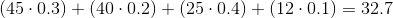# AP Statistics : How to find mean of a random variable

## Example Questions

### Example Question #1 : Random Variables

Let us suppose you are a waiter. You work your first four shifts and receive the following in tips: (1) 20, (2) 30, (3) 15, (4) 5. What is the mean amount of tips you will receive in a given day?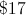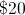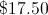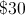Explanation:

The answer is 17.5. Simply take the values for each day, add them, and divide by the total number of days to obtain the mean: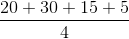### Example Question #1 : Random Variables

There arecollectable coins in a bag.areounces,areounces,areounces, andareounces. If one coin is randomly selected, what is the mean possible weight in ounces?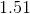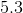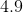Explanation:

We are required to find the mean outcome where the probability of each possible result varies--the random/weighted mean.

First, multiply each possible outcome by the probability of that outcome occurring.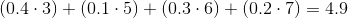### Example Question #1 : How To Find Mean Of A Random Variable

A basketball player makesof his three-point shots. If he takesthree-point shots each game, how many points per game does he score from three-point range?Explanation:

First convert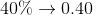.

The player's three-point shooting follows a binomial distribution with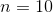and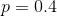.

On average, he thus makes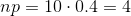three-point shots per game.

This means he averages 12 points per game from three-point range if he tries to make 10 three-pointers per game.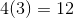### Example Question #4 : How To Find Mean Of A Random Variable

Tim samples the average plant height of potato plants for his science class and finds the following distribution (in inches):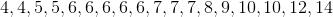Which of the following is/are true about the data?

i: the mode isii: the mean is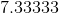iii: the median isiv: the range isi & ii

i & iii

all of the above

ii, iii & iv

i, ii & iii

i & ii

Explanation:

Analyzing the data, there are more 6s than anything else (mode), the median is betweenand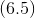, the mean is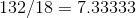, and the range is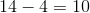### Example Question #71 : Statistical Patterns And Random Phenomena

Robert's work schedule for next week will be released today.  Robert will work either 45, 40, 25, or 12 hours.  The probabilities for each possibility are listed below:

45 hours: 0.3

40 hours: 0.2

25 hours: 0.4

12 hours: 0.1

What is the mean outcome for the number of hours that Robert will work?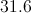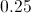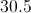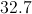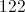Explanation:

We are required to find the mean outcome where the probability of each possible result varies--the random/weighted mean.  First, multiply each possible outcome by the probability of that outcome occurring.  Second, add these results together.